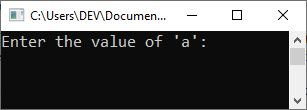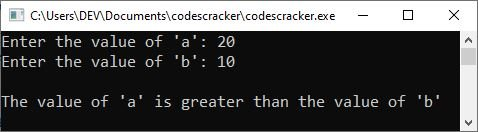# C++ if, if...else, and if...else if...else statements

The following selection statements offered by the "C++ language" allow you to select the set of instructions to carry out based on the truth value or condition of an expression. This article was written and published to cover these selection statements.

The "switch" will be covered in a separate post after the first three are covered in this one.

## C++ if statement

An "if" statement evaluates a specific condition; if the condition is true, action is taken; otherwise, action is not taken. Action can be thought of as a statement or set of statements. The if statement's syntax, or general form, is as follows:

```if(expression)
{
statement(s);
}```

Here, a statement may be a single statement, a block of statements, or nothing (in the case of an empty statement). The expression must be enclosed in parentheses. If the expression evaluates to true, i.e., a nonzero value, the statement is executed; otherwise, it is ignored. For example, the following code fragment

```if(ch == ' ')
{
spaces++;
}```

Checks whether the character variable ch contains a space; if so, the number of spaces is increased by one. Consider another example illustrating the use of an if statement:

```if(num%2==0)
cout<<"It is an even number.";```

The code fragment above shows that if the variable "num" value is completely divided by the number "2" without leaving a remainder, the text "It is an even number." will be printed on the output console.

That is, if the given condition "num%2==0" evaluates to true, then the following statement:

`cout<<"It is an even number.";`

will be executed. And if the given condition evaluates to false, then the above statement will not be executed.

Please note: If the "if" statement contains a single statement in its block, then it is your choice whether to include the curly braces. Otherwise, if the "if" block contains multiple statements, then you need to put all the statements inside a curly brace in this way:

```if(num%2==0)
{
cout<<"It is an even number.";
cout<<"\nThe value of 'num' is "<<num;
}```

### C++ if statement example program

The following example program can be considered an example program for the "if" statement in C++.

```#include<iostream>
using namespace std;
int main()
{
int a, b;

cout<<"Enter the value of 'a': ";
cin>>a;
cout<<"Enter the value of 'b': ";
cin>>b;

if(a>b)
cout<<"\nThe value of 'a' is greater than the value of 'b'";

cout<<endl;

return 0;
}```

The following snapshot shows the initial output produced by this program:Now supply the value of "a," say 20, and hit the ENTER key. Type another number, say 10 as the value of "b," and hit the ENTER key to execute the "if" statement. Based on the same input as I told you right now, here is the sample run:The above program is missing one feature: we will not receive any output if the value of "a" is less than "b." So now is the time to employ the "if...else" statement.

## C++ if-else Statement

The "if-else" statement is similar to that of the "if" statement in C++, except that, in this case, we will have another block, which is called the "else" block, whose given set of statements will be executed if the specified if's condition evaluates to false. Following is the general form of the "if-else" statement in C++:

```if(expression)
{
// set of statements to execute
// if the "expression" evaluates to true
}
else
{
// set of statements to execute
// if the "expression" evaluates to false
}```

### C++ if-else statement example program

To create an example program for the "if-else" statement, I just modified the example program given in the "if" statement's example. So here is the modified version of the previous program.

```#include<iostream>
using namespace std;
int main()
{
int a, b;

cout<<"Enter the value of 'a': ";
cin>>a;
cout<<"Enter the value of 'b': ";
cin>>b;

if(a>b)
cout<<"\nThe value of 'a' is greater than the value of 'b'";
else
cout<<"\nThe value of 'a' is less than the value of 'b'";

cout<<endl;

return 0;
}```

The sample run with user inputs of 10 and 20 as the values of "a" and "b" should exactly be:

```Enter the value of 'a': 10
Enter the value of 'b': 20

The value of 'a' is less than the value of 'b'```

Important: The placement of the semicolon is also important. In an if statement, do not put a semicolon in the line having a test condition, such as

`if (y > max) ;   // don't do this`

Caution: If you put a semicolon after the test condition, the if statement ends there. The block or statements following are no longer relevant in such cases.

## C++ if-else if-else Statement

The "if...else if...else" or "if-else if-else" statement is used when we need to execute the required block of code based on multiple conditions. That is, when we need to test multiple conditions and want to execute a single block of code, then we need an "if-else" statement. The following is the general form:

```if(expression1)
{
// block of code to execute
// if the "expression1" evaluates to true
}
else if(expression2)
{
// block of code to execute
// if the "expression2" evaluates to true
}
else if(expression3)
{
// block of code to execute
// if the "expression3" evaluates to true
}
.
.
.
else if(expressionN)
{
// block of code to execute
// if the "expressionN" evaluates to true
}
else
{
// block of code to execute
// if all expression's condition evaluates to false
}```

Starting with "expression1," the condition of each expression will be evaluated. If any expression evaluates to true, then its block of code will be executed, and all the remaining expressions in the "else if" statement, including the "else," will be skipped. For example:

```#include<iostream>
using namespace std;
int main()
{
int a, b;

cout<<"Enter the value of 'a': ";
cin>>a;
cout<<"Enter the value of 'b': ";
cin>>b;

if(a>b)
cout<<"\nThe value of 'a' is greater than the value of 'b'";
else if(a<b)
cout<<"\nThe value of 'a' is less than the value of 'b'";
else
cout<<"\nBoth values are equal";

cout<<endl;

return 0;
}```

Following is the sample run of the above program with user inputs of 10 and 10 as the values of "a" and "b":

```Enter the value of 'a': 10
Enter the value of 'b': 10

Both values are equal```

#### More Examples

Here are some more C++ programs listed, that you may like:

C++ Quiz

« Previous Tutorial Next Tutorial »